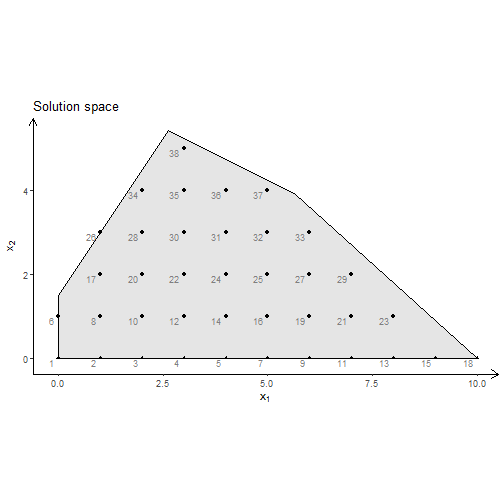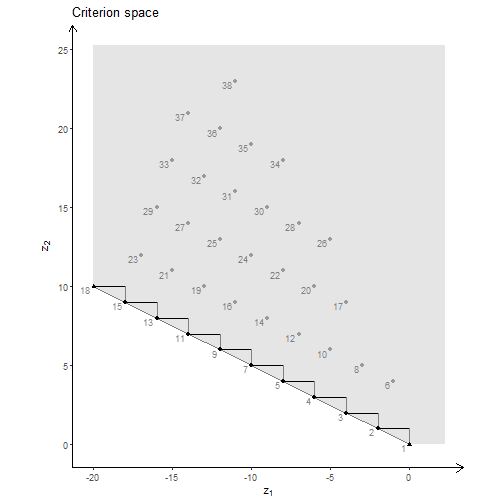I recent released a small package gMOIP which can make 2D plots of linear and integer programming models (LP/IP). With the package you can make plots of the feasible region (or solution space) of an LP, visualize the integer points inside the region and the iso profit curve. Moreover, can also make a plot of a bi-objective criterion space and the non-dominated (Pareto) set. Figures are prepared for LaTeX and can automatically be transformed to TikZ using tikzDevice.

You can install the latest stable release from CRAN:

install.packages("gMOIP")


Alternatively, install the latest development version from GitHub:

install.packages("devtools")
devtools::install_github("relund/gMOIP")


Let us have a look at some examples. First we specify the feasible region and calculate the corner points of the polytope and the integer points inside the feasible region.

# Feasible region Ax<=b, x>=0
A <- matrix(c(9,10,2,4,-3,2), ncol = 2, byrow = TRUE)
b <- c(90,27,3)
# Corner points of the polytope
cPoints<-cornerPoints(A, b)
# Integer points in the polytope
iPoints<-integerPoints(A, b)


It is now easy to make different plots of the polytope using plotPolytope:

# Plot of the polytope
p1 <- plotPolytope(cPoints) + ggtitle("Feasible region only")
p2 <- plotPolytope(cPoints, cPoints, iso = c(7.75, 10), crit = "max") +
ggtitle("Solution LP (max)")
p3 <- plotPolytope(cPoints, iPoints, iso = c(7.75, 10), crit = "max") +
ggtitle("Solution IP (max)")
p4 <- plotPolytope(cPoints, iPoints, iso = c(3,-3), crit = "min") +
ggtitle("Solution IP (min)")
grid.arrange(p1, p2, p3, p4, nrow = 2)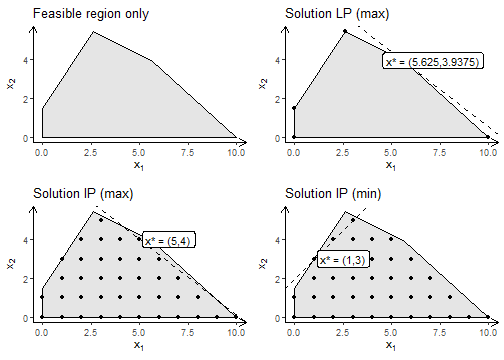You may also create a tikz file of the plot for LaTeX using:

library(tikzDevice)
tikz(file = "plot_polytope.tex", standAlone=F, width = 7, height = 6)
plotPolytope(cPoints, iPoints, iso = c(3,-3), crit = "min", latex = TRUE)
dev.off()


If you consider a bi-objective IP problem the solution space can be visualized using plotCriterion. First, consider model $\min\{ \left( (-1,1)x, (1,-1)x\right) | Ax\leq b, x\geq 0, x \text{ integer} \}$. Note that solutions and criterion points are linked using labels.

zPoints<-criterionPoints(iPoints, c1 = c(-1, 1), c2 = c(1, -1), crit = "min")
plotPolytope(cPoints, zPoints, showLbl = TRUE) + ggtitle("Solution space")
plotCriterion(zPoints, addHull = TRUE, addTriangles = TRUE, crit = "min", showLbl = TRUE) +
ggtitle("Criterion space")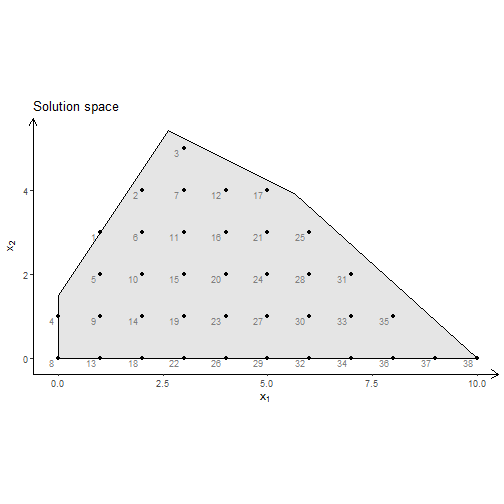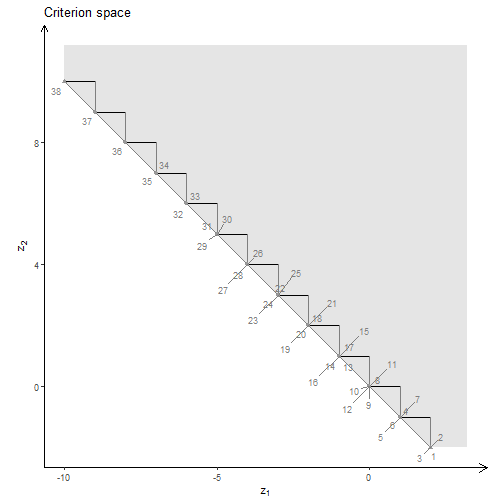Next, consider model $\max\{ \left( (2,-1)x, (1,4)x\right) | Ax\leq b, x\geq 0, x \text{ integer} \}$. Here solutions corresponding to a non-dominated point is visualized in the solution space using a different shape.

zPoints<-criterionPoints(iPoints, c1 = c(-2, -1), c2 = c(1, 4), crit = "max")
plotPolytope(cPoints, zPoints, showLbl = TRUE, shape = zPoints$nD) + ggtitle("Solution space") plotCriterion(zPoints, addHull = TRUE, addTriangles = TRUE, crit = "max", showLbl = TRUE) + ggtitle("Criterion space")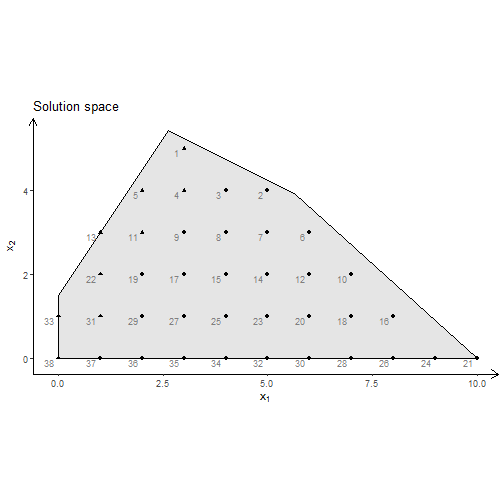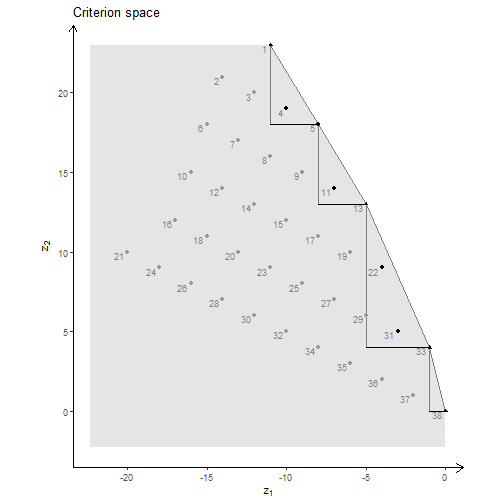Finally, consider model $\min\{ \left( (2,-1)x, (1,4)x\right) | Ax\leq b, x\geq 0, x \text{ integer} \}$ zPoints<-criterionPoints(iPoints, c1 = c(-2, -1), c2 = c(1, 4), crit = "min") plotPolytope(cPoints, zPoints, showLbl = TRUE, shape = zPoints$nD) + ggtitle("Solution space")
plotCriterion(zPoints, addHull = TRUE, addTriangles = TRUE, crit = "min", showLbl = TRUE) +
ggtitle("Criterion space")